• 梯度下降是迭代法的一种,可以用于求解最小二乘问题(线性和非线性都可以)。在求解机器学习算法的模型参数，即无...在机器学习中，基于基本的梯度下降法发展了两种梯度下降方法，分别为随机梯度下降法和批量梯度下降法
• 详细来讲讲梯度下降算法的原理，感受数学和程序的魅力吧！！


博文目录
1. 概述2. 梯度下降算法2.1 场景假设2.2 梯度下降2.2.1 微分2.2.2 梯度
2.3 数学解释2.3.1 α2.3.2 梯度要乘以一个负号

3. 实例3.1 单变量函数的梯度下降3.2 多变量函数的梯度下降
4. 代码实现4. 1 场景分析4. 2 代码
5. 小结

1. 概述
2. 梯度下降算法
2.1 场景假设
梯度下降法的基本思想可以类比为一个下山的过程。 假设这样一个场景：一个人被困在山上，需要从山上下来(找到山的最低点，也就是山谷)。但此时山上的浓雾很大，导致可视度很低；因此，下山的路径就无法确定，必须利用自己周围的信息一步一步地找到下山的路。这个时候，便可利用梯度下降算法来帮助自己下山。怎么做呢，首先以他当前的所处的位置为基准，寻找这个位置最陡峭的地方，然后朝着下降方向走一步，然后又继续以当前位置为基准，再找最陡峭的地方，再走直到最后到达最低处；同理上山也是如此，只是这时候就变成梯度上升算法了2.2 梯度下降
梯度下降的基本过程就和下山的场景很类似。
首先，我们有一个可微分的函数。这个函数就代表着一座山。我们的目标就是找到这个函数的最小值，也就是山底。根据之前的场景假设，最快的下山的方式就是找到当前位置最陡峭的方向，然后沿着此方向向下走，对应到函数中，就是找到给定点的梯度 ，然后朝着梯度相反的方向，就能让函数值下降的最快！因为梯度的方向就是函数之变化最快的方向(在后面会详细解释) 所以，我们重复利用这个方法，反复求取梯度，最后就能到达局部的最小值，这就类似于我们下山的过程。而求取梯度就确定了最陡峭的方向，也就是场景中测量方向的手段。那么为什么梯度的方向就是最陡峭的方向呢？接下来，我们从微分开始讲起：
2.2.1 微分
看待微分的意义，可以有不同的角度，最常用的两种是：
函数图像中，某点的切线的斜率函数的变化率 几个微分的例子：
1.单变量的微分，函数只有一个变量时

d

(

x

2

)

d

x

=

2

x

\frac{d(x^2)}{dx}=2x

d

(

−

2

y

5

)

d

y

=

−

10

y

4

\frac{d(-2y^5)}{dy}=-10y^4

d

(

5

−

θ

)

2

d

θ

=

−

2

(

5

−

θ

)

\frac{d(5-\theta )^2}{d\theta}=-2(5-\theta)

2.多变量的微分，当函数有多个变量的时候，即分别对每个变量进行求微分

∂

∂

x

(

x

2

y

2

)

=

2

x

y

2

\frac{\partial}{\partial x}(x^2y^2) = 2xy^2

∂

∂

y

(

−

2

y

5

+

z

2

)

=

−

10

y

4

\frac{\partial}{\partial y}(-2y^5+z^2) = -10y^4

∂

∂

θ

2

(

5

θ

1

+

2

θ

2

−

12

θ

3

)

=

2

\frac{\partial}{\partial \theta_{2}}(5\theta_{1} + 2\theta_{2} - 12\theta_{3}) = 2

∂

∂

θ

2

(

0.55

−

(

5

θ

1

+

2

θ

2

−

12

θ

3

)

)

=

−

2

\frac{\partial}{\partial \theta_{2}}(0.55 - (5\theta_{1} + 2\theta_{2} - 12\theta_{3})) = -2

2.2.2 梯度
梯度实际上就是多变量微分的一般化。 下面这个例子：

J

(

Θ

)

=

0.55

−

(

5

θ

1

+

2

θ

2

−

12

θ

3

)

J(\Theta ) = 0.55 - (5\theta_{1} + 2\theta_{2} - 12\theta_{3})

▽

J

(

Θ

)

=

<

∂

J

∂

θ

1

,

∂

J

∂

θ

2

,

∂

J

∂

θ

3

>

=

(

−

5

,

−

2

,

12

)

\triangledown J(\Theta ) = \left < \frac{\partial J}{\partial \theta_{1}}, \frac{\partial J}{\partial \theta_{2}},\frac{\partial J}{\partial \theta_{3}} \right > =(-5,-2,12)

我们可以看到，梯度就是分别对每个变量进行微分，然后用逗号分割开，梯度是用<>包括起来，说明梯度其实一个向量。
梯度是微积分中一个很重要的概念，之前提到过梯度的意义
在单变量的函数中，梯度其实就是函数的微分，代表着函数在某个给定点的切线的斜率在多变量函数中，梯度是一个向量，向量有方向，梯度的方向就指出了函数在给定点的上升最快的方向
这也就说明了为什么我们需要千方百计的求取梯度！我们需要到达山底，就需要在每一步观测到此时最陡峭的地方，梯度就恰巧告诉了我们这个方向。梯度的方向是函数在给定点上升最快的方向，那么梯度的反方向就是函数在给定点下降最快的方向，这正是我们所需要的。所以我们只要沿着梯度的方向一直走，就能走到局部的最低点！
2.3 数学解释
首先给出数学公式：

Θ

1

=

Θ

0

+

α

▽

J

(

Θ

)

→

e

v

a

l

u

a

t

e

d

a

t

Θ

0

{\color{Red} \Theta^1} = {\color{Blue} \Theta^0} + {\color{Green} \alpha} {\color{Purple} \triangledown J(\Theta)}\rightarrow evaluated at \Theta^0

此公式的意义是：J是关于Θ的一个函数，我们当前所处的位置为Θ0点，要从这个点走到J的最小值点，也就是山底。首先我们先确定前进的方向，也就是梯度的反向，然后走一段距离的步长，也就是α，走完这个段步长，就到达了Θ1这个点！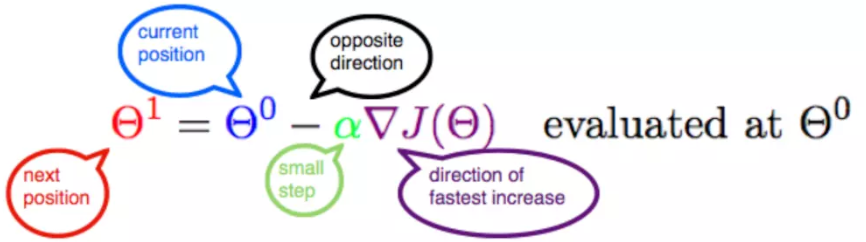2.3.1 α
α在梯度下降算法中被称作为学习率或者步长，意味着我们可以通过α来控制每一步走的距离，以保证不要步子跨的太大扯着蛋，哈哈，其实就是不要走太快，错过了最低点。同时也要保证不要走的太慢，导致太阳下山了，还没有走到山下。所以α的选择在梯度下降法中往往是很重要的！α不能太大也不能太小，太小的话，可能导致迟迟走不到最低点，太大的话，会导致错过最低点！
2.3.2 梯度要乘以一个负号
梯度前加一个负号，就意味着朝着梯度相反的方向前进！我们在前文提到，梯度的方向实际就是函数在此点上升最快的方向！而我们需要朝着下降最快的方向走，自然就是负的梯度的方向，所以此处需要加上负号；那么如果时上坡，也就是梯度上升算法，当然就不需要添加负号了。
3. 实例
我们已经基本了解了梯度下降算法的计算过程，那么我们就来看几个梯度下降算法的小实例，首先从单变量的函数开始，然后介绍多变量的函数。
3.1 单变量函数的梯度下降
我们假设有一个单变量的函数

J

(

θ

)

=

θ

2

J(\theta) = \theta^2

函数的微分，直接求导就可以得到

J

′

(

θ

)

=

2

θ

J'(\theta) = 2\theta

初始化，也就是起点，起点可以随意的设置，这里设置为1

θ

0

=

1

\theta^0 = 1

学习率也可以随意的设置，这里设置为0.4

α

=

0.4

\alpha = 0.4

根据梯度下降的计算公式

Θ

1

=

Θ

0

+

α

▽

J

(

Θ

)

→

e

v

a

l

u

a

t

e

d

a

t

Θ

0

{\color{Red} \Theta^1} = {\color{Blue} \Theta^0} + {\color{Green} \alpha} {\color{Purple} \triangledown J(\Theta)}\rightarrow evaluated at \Theta^0

我们开始进行梯度下降的迭代计算过程：

θ

0

=

1

\theta^0 = 1

θ

1

=

θ

0

−

α

∗

J

′

(

θ

0

)

=

1

−

0.4

∗

2

=

0.2

\theta^1 = \theta^0 - \alpha*J'(\theta^0)=1 - 0.4*2 = 0.2

θ

2

=

θ

1

−

α

∗

J

′

(

θ

1

)

=

0.2

−

0.4

∗

0.4

=

0.04

\theta^2 = \theta^1 - \alpha*J'(\theta^1)= 0.2 - 0.4*0.4=0.04

θ

3

=

0.008

\theta^3 = 0.008

θ

4

=

0.0016

\theta^4 = 0.0016

如图，经过四次的运算，也就是走了四步，基本就抵达了函数的最低点，也就是山底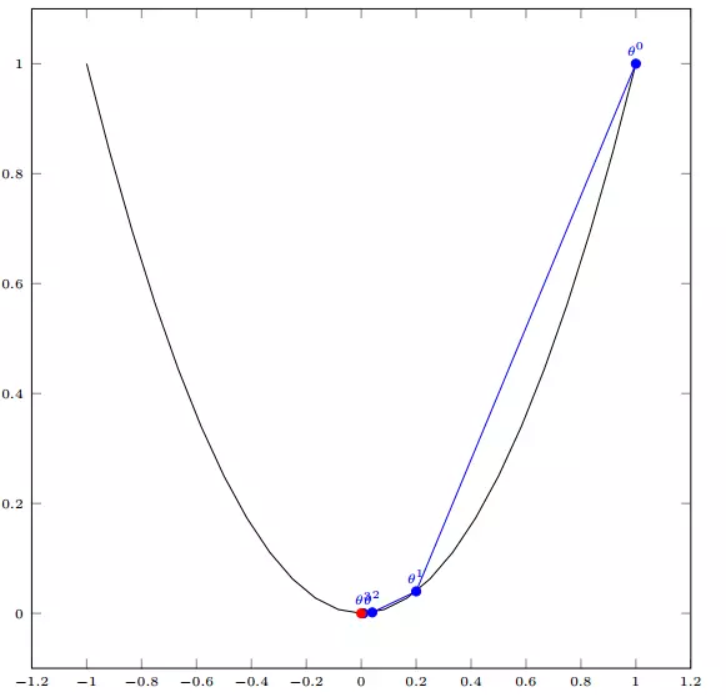3.2 多变量函数的梯度下降
我们假设有一个目标函数

J

(

Θ

)

=

θ

1

2

+

θ

2

2

J(\Theta) = \theta_{1}^2 + \theta_{2}^2

现在要通过梯度下降法计算这个函数的最小值。我们通过观察就能发现最小值其实就是 (0，0)点。但是接下来，我们会从梯度下降算法开始一步步计算到这个最小值！ 我们假设初始的起点为：

Θ

0

=

(

1

,

3

)

\Theta^0 = (1, 3)

初始的学习率为：

α

=

0.1

\alpha = 0.1

函数的梯度为：

▽

J

(

Θ

)

=

<

2

θ

1

,

2

θ

2

>

\triangledown J(\Theta ) = \left < 2\theta_{1},2\theta_{2} \right >

进行多次迭代：

Θ

0

=

(

1

,

3

)

\Theta^0 = (1, 3)

Θ

1

=

Θ

0

−

α

▽

J

(

Θ

)

=

(

1

,

3

)

−

0.1

∗

(

2

,

6

)

=

(

0.8

,

2.4

)

\Theta^1 = \Theta^0 - \alpha\triangledown J(\Theta ) = (1,3) - 0.1*(2, 6)=(0.8, 2.4)

Θ

2

=

(

0.8

,

2.4

)

−

0.1

∗

(

1.6

,

4.8

)

=

(

0.64

,

1.92

)

\Theta^2 = (0.8, 2.4) - 0.1*(1.6, 4.8)=(0.64, 1.92)

Θ

3

=

(

0.5124

,

1.536

)

\Theta^3 =(0.5124, 1.536)

Θ

4

=

(

0.4096

,

1.228800000000001

)

\Theta^4 =(0.4096, 1.228800000000001)

⋮

\vdots

Θ

10

=

(

0.1073741824000003

,

0.32212254720000005

)

\Theta^{10} =(0.1073741824000003, 0.32212254720000005)

⋮

\vdots

Θ

50

=

(

1.141798154164342

e

−

05

,

3.42539442494306

e

−

05

)

\Theta^{50} =(1.141798154164342e^{-05}, 3.42539442494306e^{-05})

⋮

\vdots

Θ

100

=

(

1.6296287810675902

e

−

10

,

4.8888886343202771

e

−

10

)

\Theta^{100} =(1.6296287810675902e^{-10}, 4.8888886343202771e^{-10})

我们发现，已经基本靠近函数的最小值点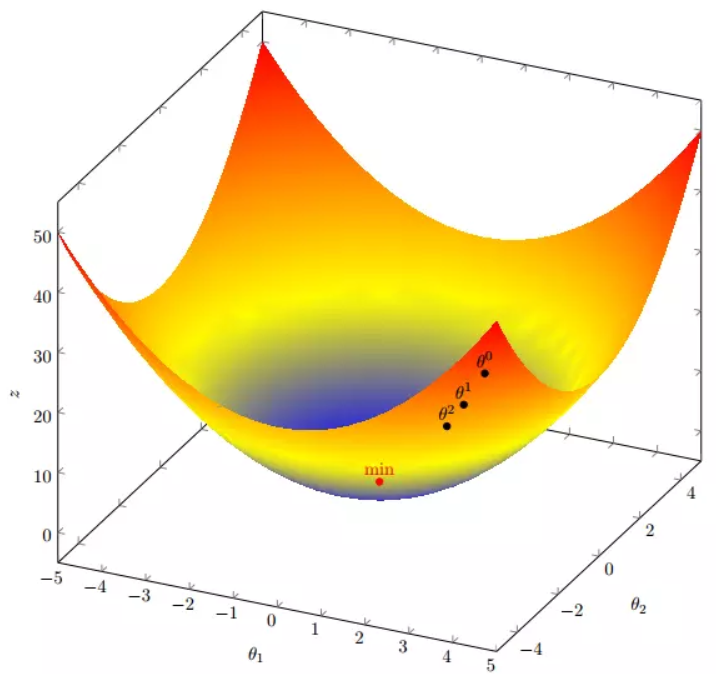4. 代码实现
4. 1 场景分析
下面我们将用python实现一个简单的梯度下降算法。场景是一个简单的线性回归的例子：假设现在我们有一系列的点，如下图所示：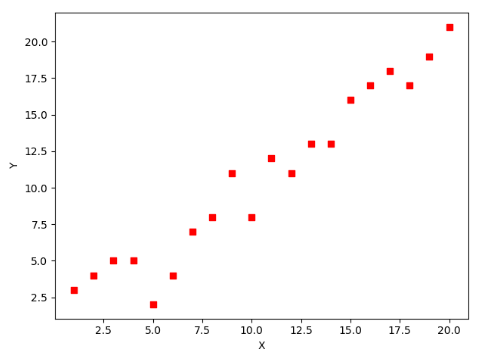我们将用梯度下降法来拟合出这条直线！
首先，我们需要定义一个代价函数，在此我们选用均方误差代价函数（也称平方误差代价函数）

J

(

Θ

)

=

1

2

m

∑

i

=

1

m

(

h

θ

(

x

(

i

)

)

−

y

(

i

)

)

2

J(\Theta) = \frac{1}{2m}\sum_{i=1}^{m}(h_{\theta}(x^{(i)})-y^{(i)})^2

此公式中
m是数据集中数据点的个数，也就是样本数½是一个常量，这样是为了在求梯度的时候，二次方乘下来的2就和这里的½抵消了，自然就没有多余的常数系数，方便后续的计算，同时对结果不会有影响y 是数据集中每个点的真实y坐标的值，也就是类标签h 是我们的预测函数（假设函数），根据每一个输入x，根据Θ 计算得到预测的y值，即

h

Θ

(

x

(

i

)

)

=

Θ

0

+

Θ

1

x

1

(

i

)

h_{\Theta}(x^{(i)}) = \Theta_{0} + \Theta_{1}x_{1}^{(i)}

我们可以根据代价函数看到，代价函数中的变量有两个，所以是一个多变量的梯度下降问题，求解出代价函数的梯度，也就是分别对两个变量进行微分

▽

J

(

Θ

)

=

<

δ

J

δ

Θ

0

,

δ

J

δ

Θ

1

>

\triangledown J(\Theta ) = \left < \frac{\delta J}{\delta \Theta_{0}}, \frac{\delta J}{\delta \Theta_{1}} \right >

δ

J

δ

Θ

0

=

1

m

∑

i

=

1

m

(

h

Θ

(

x

(

i

)

)

−

y

(

i

)

)

\frac{\delta J}{\delta \Theta_{0}} = \frac{1}{m}\sum_{i=1}^{m}(h_{\Theta}(x^{(i)})-y^{(i)})

δ

J

δ

Θ

1

=

1

m

∑

i

=

1

m

(

h

Θ

(

x

(

i

)

)

−

y

(

i

)

)

x

1

(

i

)

\frac{\delta J}{\delta \Theta_{1}} = \frac{1}{m}\sum_{i=1}^{m}(h_{\Theta}(x^{(i)})-y^{(i)})x_{1}^{(i)}

明确了代价函数和梯度，以及预测的函数形式。我们就可以开始编写代码了。但在这之前，需要说明一点，就是为了方便代码的编写，我们会将所有的公式都转换为矩阵的形式，python中计算矩阵是非常方便的，同时代码也会变得非常的简洁。 为了转换为矩阵的计算，我们观察到预测函数的形式

h

Θ

(

x

(

i

)

)

=

Θ

0

+

Θ

1

x

(

i

)

h_{\Theta}(x^{(i)}) = \Theta_{0} + \Theta_{1}x^{(i)}

我们有两个变量，为了对这个公式进行矩阵化，我们可以给每一个点x增加一维，这一维的值固定为1，这一维将会乘到Θ0上。这样就方便我们统一矩阵化的计算

(

x

1

(

i

)

,

y

(

i

)

)

→

(

x

0

(

i

)

,

x

1

(

i

)

,

y

(

i

)

)

w

i

t

h

x

0

(

i

)

=

1

∀

i

(x_{1}^{(i)},y^{(i)})\rightarrow (x_{0}^{(i)},x_{1}^{(i)},y^{(i)}) with x_{0}^{(i)} = 1 \forall _{i}

然后我们将代价函数和梯度转化为矩阵向量相乘的形式

J

(

Θ

)

=

1

2

m

(

X

Θ

−

y

⃗

)

T

(

X

Θ

−

y

⃗

)

J(\Theta) = \frac{1}{2m}(X\Theta - \vec{y})^{T}(X\Theta - \vec{y})

▽

J

(

Θ

)

=

1

m

X

T

(

X

Θ

−

y

⃗

)

)

\triangledown J(\Theta) = \frac{1}{m}X^{T}(X\Theta - \vec{y}))

4. 2 代码
首先，我们需要定义数据集和学习率
#!/usr/bin/env python3
# -*- coding: utf-8 -*-
# @Time    : 2019/1/21 21:06
# @Author  : Arrow and Bullet
# @Software: PyCharm
# @Blog    ：https://blog.csdn.net/qq_41800366

from numpy import *

# 数据集大小 即20个数据点
m = 20
# x的坐标以及对应的矩阵
X0 = ones((m, 1))  # 生成一个m行1列的向量，也就是x0，全是1
X1 = arange(1, m+1).reshape(m, 1)  # 生成一个m行1列的向量，也就是x1，从1到m
X = hstack((X0, X1))  # 按照列堆叠形成数组，其实就是样本数据
# 对应的y坐标
y = np.array([
3, 4, 5, 5, 2, 4, 7, 8, 11, 8, 12,
11, 13, 13, 16, 17, 18, 17, 19, 21
]).reshape(m, 1)
# 学习率
alpha = 0.01

接下来我们以矩阵向量的形式定义代价函数和代价函数的梯度
# 定义代价函数
def cost_function(theta, X, Y):
diff = dot(X, theta) - Y  # dot() 数组需要像矩阵那样相乘，就需要用到dot()
return (1/(2*m)) * dot(diff.transpose(), diff)

# 定义代价函数对应的梯度函数
diff = dot(X, theta) - Y
return (1/m) * dot(X.transpose(), diff)

最后就是算法的核心部分，梯度下降迭代计算
# 梯度下降迭代
theta = array([1, 1]).reshape(2, 1)
theta = theta - alpha * gradient
return theta

print('optimal:', optimal)
print('cost function:', cost_function(optimal, X, Y))

当梯度小于1e-5时，说明已经进入了比较平滑的状态，类似于山谷的状态，这时候再继续迭代效果也不大了，所以这个时候可以退出循环！ 运行代码，计算得到的结果如下：
print('optimal:', optimal)  # 结果 [[0.51583286][0.96992163]]
print('cost function:', cost_function(optimal, X, Y))  # 1.014962406233101

通过matplotlib画出图像，
# 根据数据画出对应的图像
def plot(X, Y, theta):
import matplotlib.pyplot as plt
ax = plt.subplot(111)  # 这是我改的
ax.scatter(X, Y, s=30, c="red", marker="s")
plt.xlabel("X")
plt.ylabel("Y")
x = arange(0, 21, 0.2)  # x的范围
y = theta + theta*x
ax.plot(x, y)
plt.show()

plot(X1, Y, optimal)

所拟合出的直线如下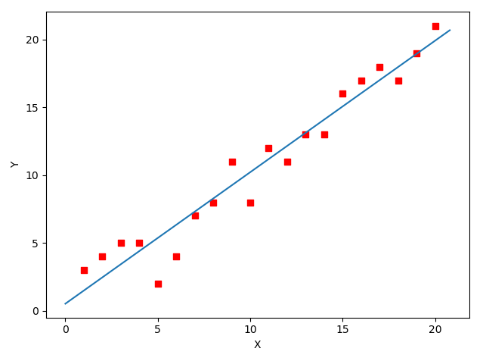全部代码如下，大家有兴趣的可以复制下来跑一下看一下结果：
#!/usr/bin/env python3
# -*- coding: utf-8 -*-
# @Time    : 2019/1/21 21:06
# @Author  : Arrow and Bullet
# @Software: PyCharm
# @Blog    ：https://blog.csdn.net/qq_41800366

from numpy import *

# 数据集大小 即20个数据点
m = 20
# x的坐标以及对应的矩阵
X0 = ones((m, 1))  # 生成一个m行1列的向量，也就是x0，全是1
X1 = arange(1, m+1).reshape(m, 1)  # 生成一个m行1列的向量，也就是x1，从1到m
X = hstack((X0, X1))  # 按照列堆叠形成数组，其实就是样本数据
# 对应的y坐标
Y = array([
3, 4, 5, 5, 2, 4, 7, 8, 11, 8, 12,
11, 13, 13, 16, 17, 18, 17, 19, 21
]).reshape(m, 1)
# 学习率
alpha = 0.01

# 定义代价函数
def cost_function(theta, X, Y):
diff = dot(X, theta) - Y  # dot() 数组需要像矩阵那样相乘，就需要用到dot()
return (1/(2*m)) * dot(diff.transpose(), diff)

# 定义代价函数对应的梯度函数
diff = dot(X, theta) - Y
return (1/m) * dot(X.transpose(), diff)

# 梯度下降迭代
theta = array([1, 1]).reshape(2, 1)
theta = theta - alpha * gradient
return theta

print('optimal:', optimal)
print('cost function:', cost_function(optimal, X, Y))

# 根据数据画出对应的图像
def plot(X, Y, theta):
import matplotlib.pyplot as plt
ax = plt.subplot(111)  # 这是我改的
ax.scatter(X, Y, s=30, c="red", marker="s")
plt.xlabel("X")
plt.ylabel("Y")
x = arange(0, 21, 0.2)  # x的范围
y = theta + theta*x
ax.plot(x, y)
plt.show()

plot(X1, Y, optimal)

5. 小结
至此，就基本介绍完了梯度下降法的基本思想和算法流程，并且用python实现了一个简单的梯度下降算法拟合直线的案例！ 最后，我们回到文章开头所提出的场景假设: 这个下山的人实际上就代表了反向传播算法，下山的路径其实就代表着算法中一直在寻找的参数Θ，山上当前点的最陡峭的方向实际上就是代价函数在这一点的梯度方向，场景中观测最陡峭方向所用的工具就是微分 。在下一次观测之前的时间就是有我们算法中的学习率α所定义的。 可以看到场景假设和梯度下降算法很好的完成了对应！
本文部分内容来自一位前辈，非常感谢分享！谢谢！
展开全文• 梯度下降PPT，从优化算法的发展历史来看梯度下降及其变体在机器学习中的作用，从最基础的优化讲起，逐渐深入，最后以简单的例子区分梯度下降家族各个成员。
• 在求解机器学习算法的模型参数，即无约束优化问题时，梯度下降法是最常采用的方法之一。 多元函数的图像显示 方程为z=x1 ^2 + 2 * x2 ^2 – 4 * x1- 2 * x1 * x2 import numpy as np import matplotlib.pyplot as ...
• 自己学习吴恩达机器学习的总结，主要是关于梯度下降算法的一些推导与介绍，以及解释了，吴恩达课中没有解释推导的内容。
• 本文实例为大家分享了python批量梯度下降算法的具体代码，供大家参考，具体内容如下 问题： 将拥有两个自变量的二阶函数绘制到空间坐标系中，并通过批量梯度下降算法找到并绘制其极值点 大体思路： 首先，根据题意...
• 这是一个matlab对梯度下降的实现，模拟的是x^2+y^2最小值的取得
• 损失使用平方函数，简单的线性模型 y = theta1 + theta2 * x
• 梯度下降算法代码及详细解释（非常易懂）Matlab代码，有详细图文解释，适合小白，全面介绍算法原理和公式推导
• c#实现梯度下降算法逻辑回归c#实现梯度下降算法逻辑回归c#实现梯度下降算法逻辑回归
• 局部优化算法之一 梯度下降法 李金屏 济南大学信息科学与工程学院 2006年9月 优选 * 优选 * 优化算法和运筹学 优化算法 许多实际问题利用数学建模的方法得到下面常规的优化形式 min f(x)s.t. g(x) 0, xD. 其中x是一...
• 最优化算法python实现篇（4）——无约束多维极值（梯度下降法）摘要算法简介注意事项算法适用性python实现实例运行结果算法过程可视化 摘要 本文介绍了多维无约束极值优化算法中的梯度下降法，通过python进行实现，...
• 简单解释：比如拿温度传感器来说，就是根据之前一段时间的温度数据计算下当前理论上应该测量到的温度，如果超出这个最优解的一定比例，就可以理解为突发状况了
• 梯度下降法是一种机器学习中常用的优化算法，用来找到一个函数(f)的参数(系数)的值，使成本函数(cost)最小。当参数不能解析计算时(如使用线性代数)，并且必须通过优化算法搜索时，它是最佳选择。批梯度下降法batch...

什么是梯度下降法？
梯度下降法是一种机器学习中常用的优化算法，用来找到一个函数(f)的参数(系数)的值，使成本函数(cost)最小。
当参数不能解析计算时(如使用线性代数)，并且必须通过优化算法搜索时，它是最佳选择。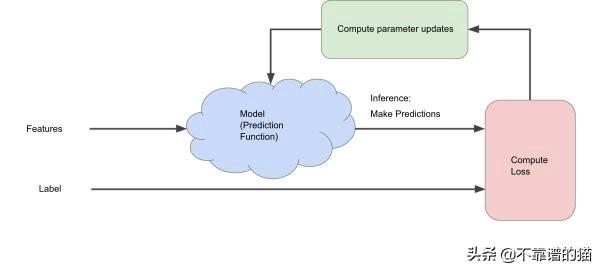批梯度下降法
batch梯度下降针对每次梯度下降迭代处理所有训练实例。如果训练样本的数量很大，那么批梯度下降在计算上将非常昂贵。因此，如果训练实例的数量很大，则批梯度下降不是首选。相反，我们更喜欢使用随机梯度下降或mini-batch梯度下降。
它是如何运作的？
由于我们需要计算整个机器学习数据集上的梯度来执行一次更新，因此批梯度下降可能非常慢，并且对于内存中不适合的数据集来说很棘手。用任意值初始化参数(说θ1=θ2=…=θn= 0)后，我们使用以下关系式计算成本函数的梯度：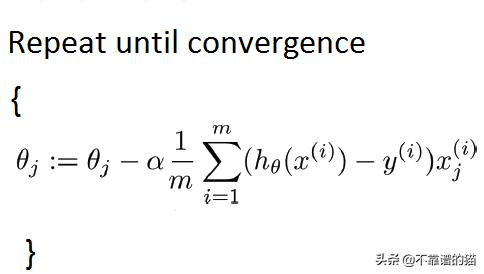其中“ m”是训练样本的数量。
如果您有1万条记录，则需要将所有记录从磁盘读入内存。在计算了一次迭代的sigma之后，我们执行了一步。然后对每个步骤重复一次。这意味着收敛需要很长时间。
因此，我们将倾向于使用其他方法。
随机梯度下降法
随机梯度下降中，每次迭代处理一个训练实例。因此，这比批梯度下降要快得多。但是，当训练样本的数量很大时，它也只处理一个样本，这可能会增加系统的开销，因为迭代的数量会非常大。
它是如何工作的？
算法的第一步是将整个训练集随机化。然后，对于每个参数的更新，我们在每次迭代中仅使用一个训练样本来计算成本函数的梯度。
由于它在每次迭代中都使用一个训练样本，因此对于较大的机器学习数据集，此方法更快。用任意值初始化参数(说θ1=θ2=…=θn= 0)后，我们使用以下关系式计算成本函数的梯度：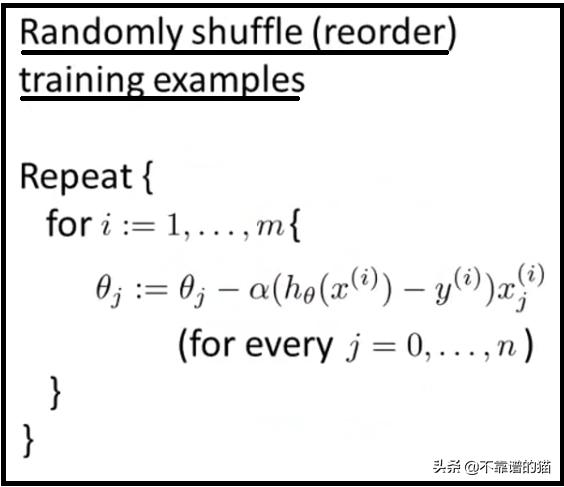其中“ m”是训练样本的数量
选择第一个训练样本并使用该样本更新参数，然后选择第二个样本，依此类推然后选择第二个训练样本，并使用该样本更新参数，以此类推。选择第三个直到n。直到达到全局最小值。
随机梯度下降法实际上不像批梯度下降法那样收敛，而是在接近全局最小值的区域内徘徊。
Mini Batch梯度下降法
Mini Batch梯度下降的速度比批梯度下降和随机梯度下降均快。在这里，每个迭代处理b个样本，其中b(m是训练样本的数量)。因此，即使训练样本数量很多，也要一次性处理b个训练样本的批次。因此，它适用于较大的训练样本，并且适用于较少的迭代次数。
如何运作
由于它是使用最广泛的算法，可以产生准确，快速的结果。
减少了参数更新的方差，这可以导致更稳定的收敛。可以利用高度优化的矩阵，从而使梯度计算非常有效。
用任意值初始化参数后，我们使用以下关系式计算成本函数的梯度：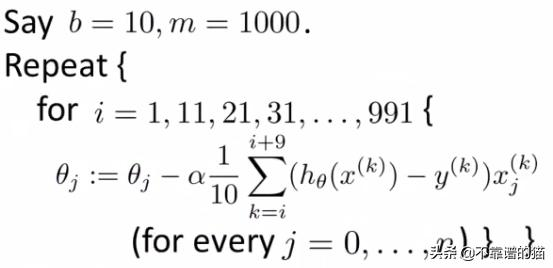alpha是学习率
常见的Mini Batch大小在50到256之间，但是会因不同的应用而有所不同。
最后
批梯度下降，Mini Batch梯度下降和随机梯度下降之间的区别是用于执行一个更新步骤的样本数。经过微调的mini-batch，其性能优于梯度下降或随机梯度下降。
基于准确度和时间消耗等参数的批量梯度下降法、小批量梯度下降法和随机梯度下降法的区别是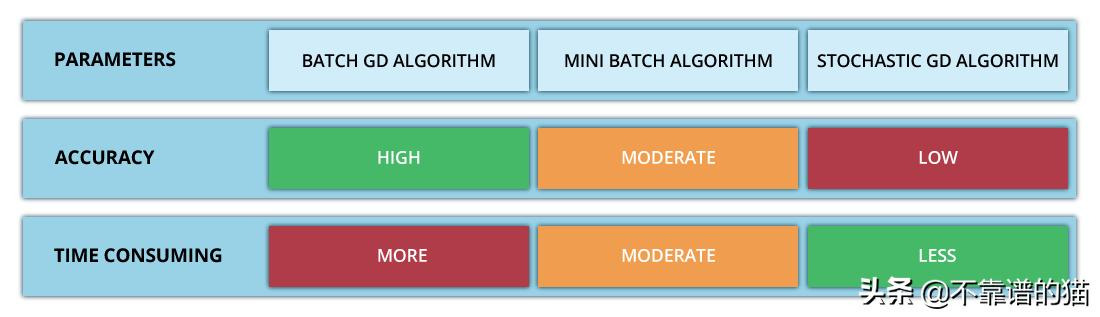展开全文• ## 梯度下降算法

千次阅读 2019-11-29 14:14:14
梯度下降法的基本思想可以类比为一个下山的过程 假设这样一个场景: 一个人被困在山上，需要从山上下来(i.e. 找到山的最低点，也就是山谷)。但此时山上的浓雾很大，导致可视度很低。 因此，下山的路径就无法确定，他...
4.梯度下降
4.1什么是梯度下降？
梯度下降法的基本思想可以类比为一个下山的过程
假设这样一个场景:
一个人被困在山上，需要从山上下来(i.e. 找到山的最低点，也就是山谷)。但此时山上的浓雾很大，导致可视度很低。
因此，下山的路径就无法确定，他必须利用自己周围的信息去找到下山的路径。这个时候，他就可以利用梯度下降算法来帮助自己下山。
具体来说就是，以他当前的所处的位置为基准，寻找这个位置最陡峭的地方，然后朝着山的高度下降的地方走，（同理，如果我们的目标是上山，也就是爬到山顶，那么此时应该是朝着最陡峭的方向往上走）。然后每走一段距离，都反复采用同一个方法，最后就能成功的抵达山谷。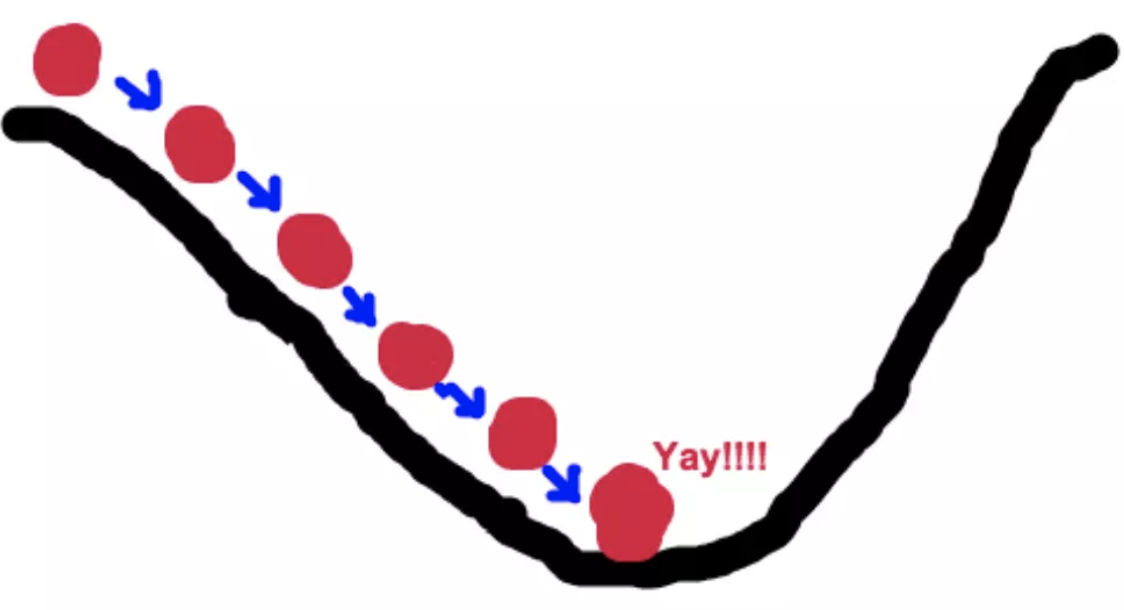梯度下降的基本过程就和下山的场景很类似。
首先，我们有一个可微分的函数。这个函数就代表着一座山。
我们的目标就是找到这个函数的最小值，也就是山底。
根据之前的场景假设，最快的下山的方式就是找到当前位置最陡峭的方向，然后沿着此方向向下走，对应到函数中，就是找到给定点的梯度 ，然后朝着梯度相反的方向，就能让函数值下降的最快！因为梯度的方向就是函数值变化最快的方向。 所以，我们重复利用这个方法，反复求取梯度，最后就能到达局部的最小值，这就类似于我们下山的过程。而求取梯度就确定了最陡峭的方向，也就是场景中测量方向的手段。
4.2 梯度的概念
梯度是微积分中一个很重要的概念
在单变量函数中，梯度其实就是函数的微分，代表着函数在某个给定点的切线的斜率；
在多变量函数中，梯度就是一个向量，向量有方向，梯度的方向就指出了函数在给定点的上升最快的方向；
这也就说明了我们为什么要千方百计是求取梯度！
4.3 梯度下降举例
1.单变量函数的梯度下降 我们假设有一个单变量的函数 :J(θ) = θ2 函数的微分:J、(θ) = 2θ 初始化，起点为： θ0 = 1 学习率：α = 0.4 我们开始进行梯度下降的迭代计算过程: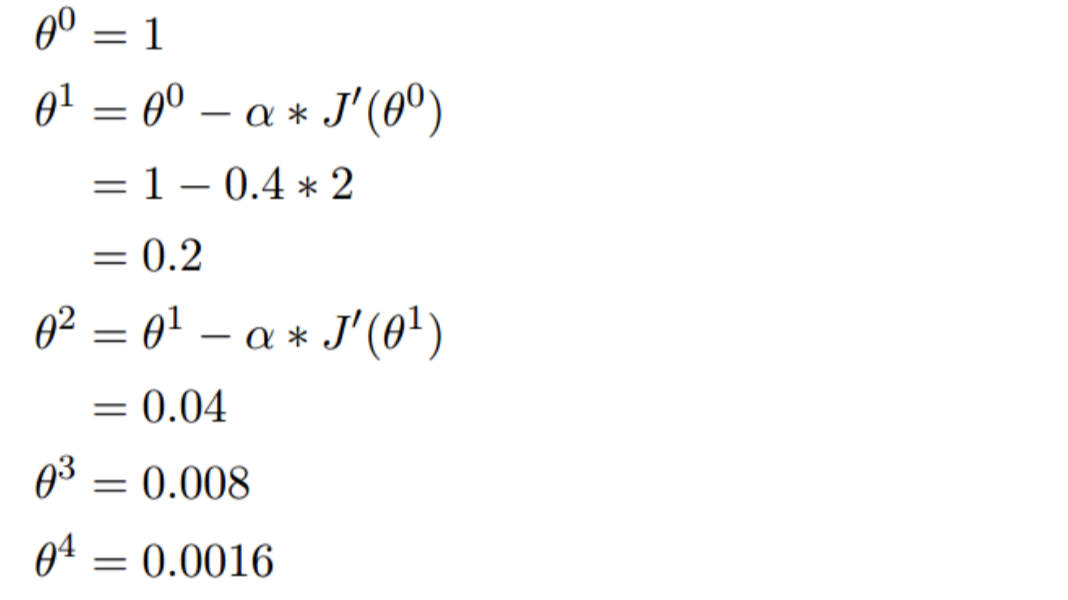如图，经过四次的运算，也就是走了四步，基本就抵达了函数的最低点，也就是山底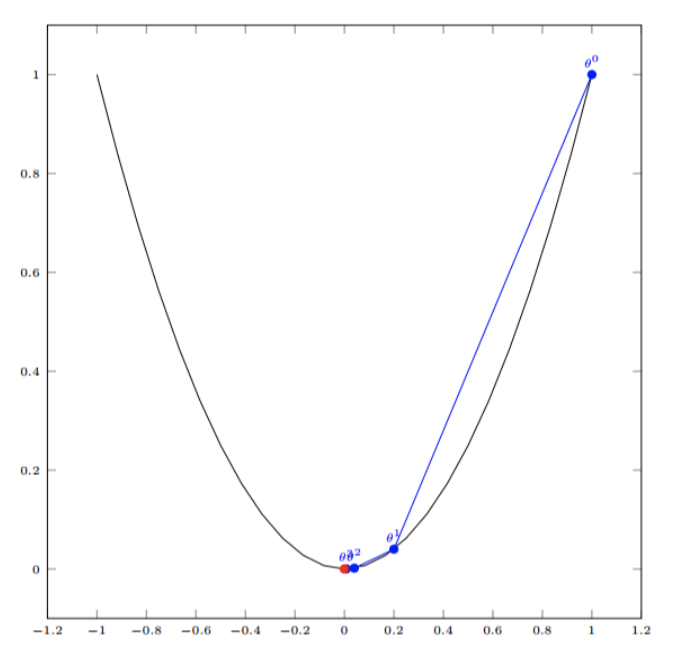2.多变量函数的梯度下降 我们假设有一个目标函数 ：:J(θ) = θ12 + θ22 现在要通过梯度下降法计算这个函数的最小值。我们通过观察就能发现最小值其实就是 (0，0)点。但是接下 来，我们会从梯度下降算法开始一步步计算到这个最小值! 我们假设初始的起点为: θ0 = (1, 3) 初始的学习率为:α = 0.1 函数的梯度为:▽:J(θ) =< 2θ1 ,2θ2> 进行多次迭代: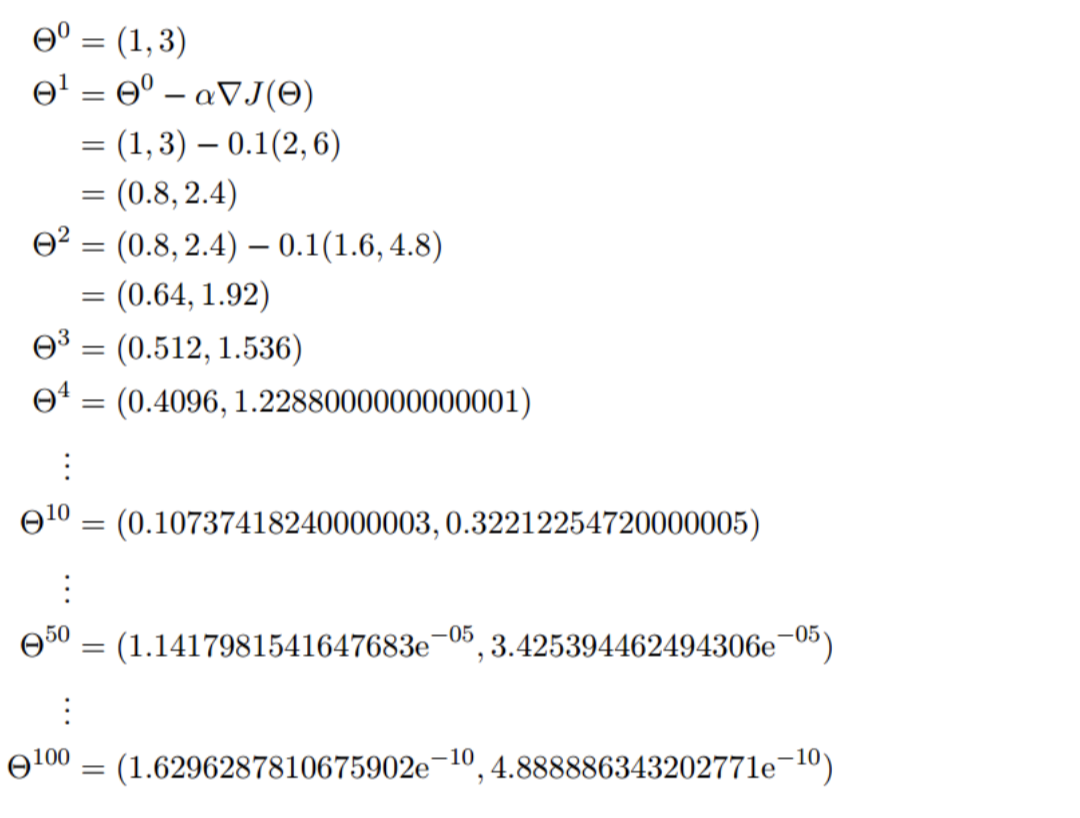我们发现，已经基本靠近函数的最小值点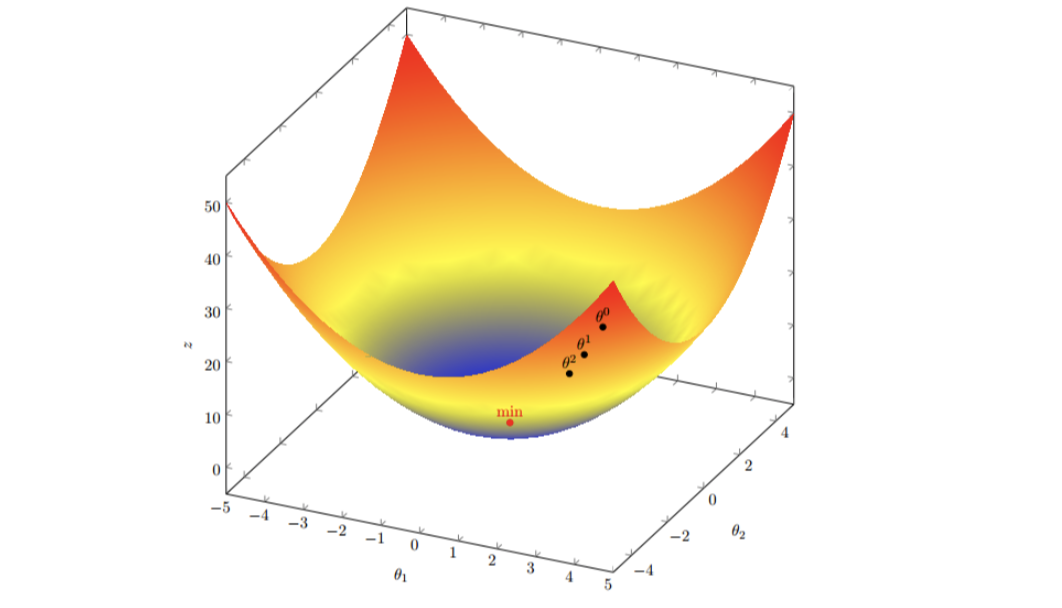4.4 梯度下降的公式1）a是什么含义？
a在梯度下降算法中被称作学习率或者步长，意味着我们可以通过a来控制每一步走的距离，以保证不要步子跨的太大，错过最低点，同时也不要走的太慢，导致效率很低。所以a的选择在梯度下降法中很重要。a不能太大也不能太小。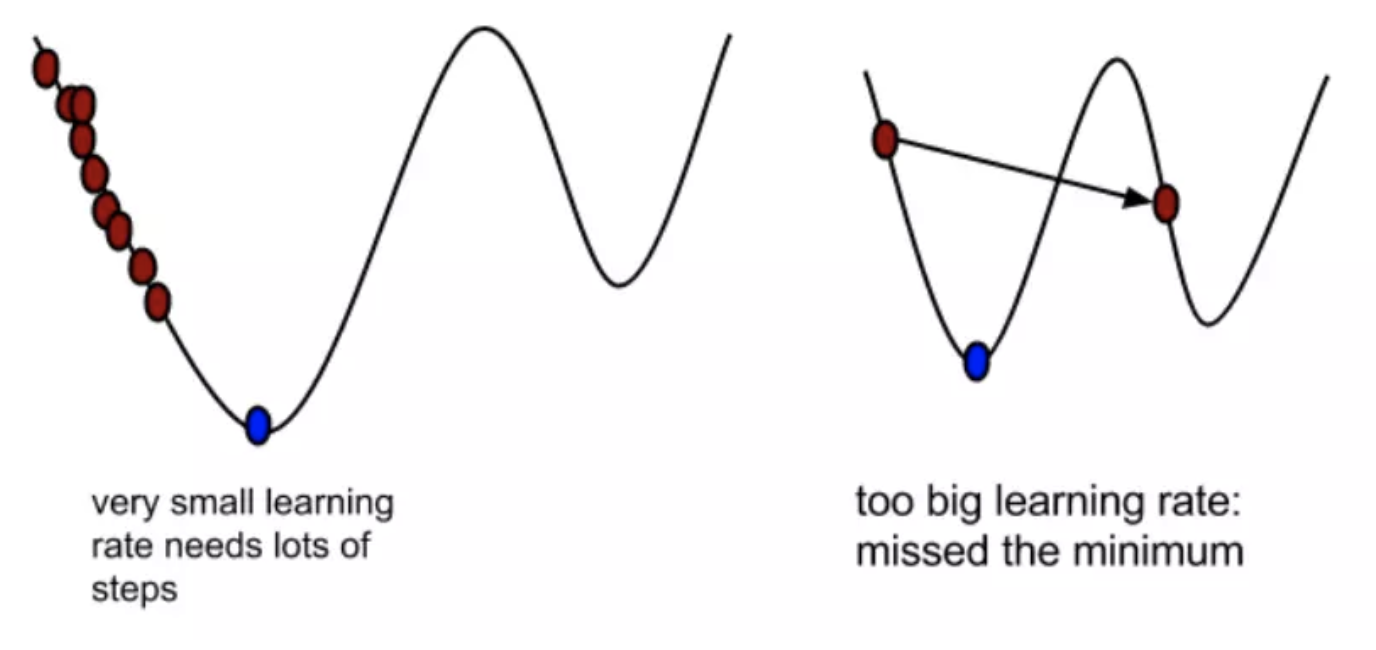2）为什么梯度要乘以一个负号？
梯度前面加上一个负号，就意味着朝梯度相反的方向走。
所以有了梯度下降这样一个优化算法，回归就有了“自动学习”的能力
5.梯度下降和正规方程的对比
梯度下降正规方程需要选择学习率不需要需要迭代求解一次运算得出特征数量较大可以使用需要计算方程，时间复杂度高O(n3)
6.算法选择依据
小规模数据
正规方程 : LinearRegression（不能解决拟合问题）岭回归 大规模数据
梯度下降法：SGDRegressor
五、梯度下降法再次介绍
常见的梯度下降算法有：
1.全梯度下降算法（FG）
计算训练集所有样本误差，对其求和再取平均值作为目标函数。
权重向量沿梯度相反方向移动，从而使当前目标函数减少的最多。
因为在执行每次更新时，我们需要在整个数据集上计算所有的梯度，所以速度会很慢，同时，无法处理超出内存限制的数据集。
批梯度下降法同样也不能在线更新模型，即在运行的过程中，不能增加新的样本。
其是在整个训练数据集上计算损失函数关于参数 θ的梯度：2.随机梯度下降法（SG）
由于FG每迭代更新一次权重都需要重新计算所有样本误差，而实际问题中经常有上亿的训练样本，故效率偏低，且容易陷入局部最优解，因此提出了随机梯度下降算法。
其每轮计算的目标函数不再是全体样本误差，而仅是单个样本误差，即每次只带入计算一个样本目标函数的梯度来更新权重，再取下一个样本重复此过程，知道损失函数值停止下降或损失函数值小于某个可以容忍的阈值。
此过程简单，高效，通常可以较好地避免更新迭代收敛到局部最优解。其迭代形式为其中，x(i)表示一条训练样本的特征值，y(i)表示一条训练样本的标签值

但是由于，SG每次只使用一个样本迭代，若遇上噪声则容易陷入局部最优解。
3.小批量梯度下降算法（mini-barch）
小批量梯度下降算法是FG和SG的这种方案，在一定程度上兼顾了以上两种方法的优点。
每次从训练样本集上随机抽取一个小样本集，在抽出来的小样本集上采用FG迭代更新权重。
被抽出的小样本集所含样本点的个数称为batch_size，通常设置为2的幂次方，更有利于GPU加速处理。
特别的，若batch_size=1，则变成了SG；若batch_size=n，则变成了FG.其迭代形式为4.随机平均梯度下降算法（SAG）
在SG方法中，虽然避开了运算成本大的问题，但对于大数据训练而言，SG效果常不尽如人意，因为每一轮梯度更新都完全与上一轮的数据和梯度无关。
随机平均梯度算法客服了这个问题，在内存中为每一个样本都维护一个旧的梯度，随机选择第i个样本来更新此样本的梯度，其他样本的梯度保持不变，然后求得所有梯度的平均值，进而更新了参数。
如此，每一轮更新仅需计算一个样本的梯度，计算成本等同于SG，但收敛速度快得多。
展开全文• 基于jupyter notebook的python编程—–利用梯度下降算法求解多元线性回归方程，并与最小二乘法求解进行精度对比目录一、梯度下降算法的基本原理1、梯度下降算法的基本原理二、题目、表格数据、以及python环境搭建1、...
• 上一篇文章讲述了梯度下降法的数学思想，趁热打铁，这篇博客笔者将使用梯度下降法完成多元线性回归，话不多说，直接开始。 我们假设我们的目标函数是长这样的： import numpy as np import pandas as pd # 读入...
• 利用梯度下降法求函数极小值，或稍作修改用梯度上升法求极大值，附带测试函数
• 这是机器学习中最常见的模型--线性回归的python实现，其中包含了最小二乘法和梯度下降法两种拟合算法
• 梯度下降法 梯度下降法的基本思想可以类比为一个下山的过程。 假设这样一个场景：一个人被困在山上，需要从山上下来(找到山的最低点，也就是山谷)。但此时山上的浓雾很大，导致可视度很低；因此，下山的路径就无法...
• 0x00 前言：机器学习方法论 在此之前，我们已经学习了分类算法：kNN算法，回归算法：线性回归。我们知道：机器学习就是需找一种函数f(x)并进行优化， 且这种函数能够做预测、分类、生成等工作。那么其实可以总结出...


0x00 前言：机器学习方法论
在此之前，我们已经学习了分类算法：kNN算法，回归算法：线性回归。我们知道：

机器学习就是需找一种函数f(x)并进行优化， 且这种函数能够做预测、分类、生成等工作。

那么其实可以总结出关于“如何找到函数f(x)”的方法论。可以看作是机器学习的“三板斧”：
第一步：定义一个函数集合(define a function set)第二步：判断函数的好坏(goodness of a function)第三步：选择最好的函数(pick the best one)
我们先把目光放在第三步上：How to pick the best one ? 我们的目标是让损失函数最小化。这就引出了下面需要介绍的方法：梯度下降是目前机器学习、深度学习解决最优化问题的算法中，最核心、应用最广的方法。
0x01 为什么需要梯度下降算法
如果我们抛开具体场景，仅从数学抽象的角度来看：每个模型都有自己的损失函数，不管是监督式学习还是非监督式学习。损失函数包含了若干个位置的模型参数，比如在多元线性回归中，损失函数：  ，其中向量表示未知的模型参数，我们就是要找到使损失函数尽可能小的参数未知模型参数。
在学习简单线性回归时，我们使用最小二乘法来求损失函数的最小值，但是这只是一个特例。在绝大多数的情况下，损失函数是很复杂的(比如逻辑回归)，根本无法得到参数估计值的表达式。因此需要一种对大多数函数都适用的方法。这就引出了“梯度算法”。
我们先了解一下梯度下降是用来做什么的?
要找到使损失函数最小化的参数，如果纯粹靠试错搜索，比如随机选择1000个值，依次作为某个参数的值，得到1000个损失值，选择其中那个让损失值最小的值，作为最优的参数值，那这样太笨了。我们需要更聪明的算法，从损失值出发，去更新参数，且要大幅降低计算次数。
0x02 什么是梯度
我们知道导数就是变化率。梯度是向量，和参数维度一样。
假设，我们有一个二元函数 。那么f的梯度为：
例如在点(1,2)，梯度∇f的取值为：
那么这个梯度有什么用呢？
在单变量的函数中，梯度其实就是函数的微分，代表着函数在某个给定点的切线的斜率 在多变量函数中，梯度是一个向量，向量有方向，梯度的方向就指出了函数在给定点的上升最快的方向
梯度指向误差值增加最快的方向，导数为0(梯度为0向量)的点，就是优化问题的解。在上面的二元函数中，点(1,2)向着(4,2)的方向就是梯度方向 为了找到这个解，我们沿着梯度的反方向进行线性搜索，从而减少误差值。每次搜索的步长为某个特定的数值，直到梯度与0向量非常接近为止。更新的点是所有点的x梯度，所有点的y梯度
0x03 理解梯度下降算法
很多求解最优化问题的方法，大多源自于模拟生活中的某个过程。比如模拟生物繁殖，得到遗传算法。模拟钢铁冶炼的冷却过程，得到退火算法。其实梯度下降算法就是模拟滚动，或者下山，在数学上可以通过函数的导数来达到这个模拟的效果。
梯度下降算法是一种思想，没有严格的定义。
3.1 场景假设

梯度下降就是从群山中山顶找一条最短的路走到山谷最低的地方。

既然是选择一个方向下山，那么这个方向怎么选？每次该怎么走？选方向在算法中是以随机方式给出的，这也是造成有时候走不到真正最低点的原因。如果选定了方向，以后每走一步，都是选择最陡的方向，直到最低点。
总结起来就一句话：随机选择一个方向，然后每次迈步都选择最陡的方向，直到这个方向上能达到的最低点。
梯度下降法的基本思想可以类比为一个下山的过程。假设这样一个场景：

一个人被困在山上，需要从山顶到山谷。但此时雾很大，看不清下山的路径。他必须利用自己周围的信息去找到下山的路径。这个时候，他就可以利用梯度下降算法来帮助自己下山。具体来说就是，以他当前的所处的位置为基准，随机选择一个方向，然后每次迈步都选择最陡的方向。然后每走一段距离，都反复采用同一个方法：如果发现脚下的路是下坡，就顺着最陡的方向走一步，如果发现脚下的路是上坡，就逆着方向走一步，最后就能成功的抵达山谷。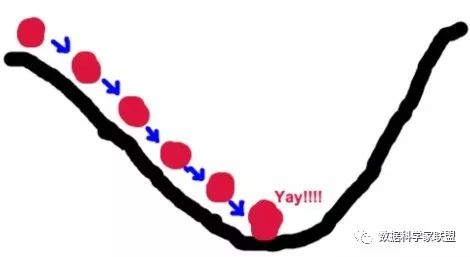3.2 数学推导
从数学的角度出发，针对损失函数L，假设选取的初始点为；现在将这个点稍微移动一点点，得到。那么根据泰勒展开式(多元函数的一阶展开式)：
设我们移动的“一点点”为 ，则我们可以得到，将泰勒展开式代入其中，我们则得到：
如果我们令移动的距离分别为： ，其中规定，则可以得到：
这就说明，我们如果按照规定的移动距离公式移动参数，那么损失函数的函数值始终是下降的，这样就达到了我们要求的“损失变小”的要求了。如果一直重复这种移动，则可以证明损失函数最终能够达到一个最小值。
那么我们就可以得到损失函数值(也就是下一步的落脚点)的迭代公式：
针对于上述公式，有一些常见的问题：
为什么要梯度要乘以一个负号？
我们已经知道：梯度的方向就是损失函数值在此点上升最快的方向，是损失增大的区域，而我们要使损失最小，因此就要逆着梯度方向走，自然就是负的梯度的方向，所以此处需要加上负号
关于参数 :
我们已经知道，梯度对应的是下山的方向，而参数 对应的是步伐的长度。在学术上，我们称之为“学习率”(learning rate)，是模型训练时的一个很重要的超参数，能直接影响算法的正确性和效率：
首先，学习率不能太大。因此从数学角度上来说，一阶泰勒公式只是一个近似的公式，只有在学习率很小，也就是很小时才成立。并且从直观上来说，如果学习率太大，那么有可能会“迈过”最低点，从而发生“摇摆”的现象(不收敛)，无法得到最低点其次，学习率又不能太小。如果太小，会导致每次迭代时，参数几乎不变化，收敛学习速度变慢，使得算法的效率降低，需要很长时间才能达到最低点。

3.3 致命问题
梯度算法有一个比较致命的问题：
从理论上，它只能保证达到局部最低点，而非全局最低点。在很多复杂函数中有很多极小值点，我们使用梯度下降法只能得到局部最优解，而不能得到全局最优解。那么对应的解决方案如下：首先随机产生多个初始参数集，即多组；然后分别对每个初始参数集使用梯度下降法，直到函数值收敛于某个值；最后从这些值中找出最小值，这个找到的最小值被当作函数的最小值。当然这种方式不一定能找到全局最优解，但是起码能找到较好的。
对于梯度下降来说，初始点的位置，也是一个超参数。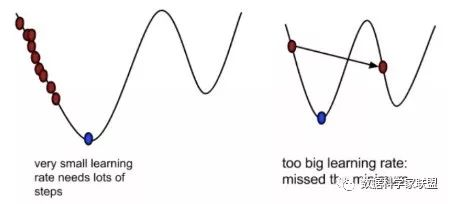0xFF 总结
在机器学习的“三板斧”中，第三步的目标是让损失函数最小化，从而引出了梯度下降法，这一目前机器学习、深度学习解决最优化问题的算法中，最核心、应用最广的方法。所谓梯度下降，是一种基于搜索的最优化方法，其作用是用来对原始模型的损失函数进行优化，找到使损失函数(局部)最小的参数。
首先对梯度下降有一个整体的印象：梯度是向量，是多元函数的导数，指向误差值增加最快的方向。我们沿着梯度的反方向进行线性搜索，从而减少误差值，是为梯度下降。然后我们通过“下山”这样的模拟场景，以及严谨的数据公式推导深刻理解了梯度下降算法，并引出了学习率的概念。最后我们给出了梯度下降方法的不足和改进方法。
相信大家看完本篇文章后，对梯度下降算法一定有了一个更加深刻的认识。如果没懂？那就再多看几遍吧(笑)展开全文• 包括单特征的样本的最小二乘法计算， 单特征样本的梯度下降法--代数版本 多特征样本的梯度下降--矩阵运算表示。 在矩阵表示的梯度下降法中运用标准差归一化（可选择注释）。 有比较详细的注释
• 牛顿法、梯度下降法原理及Python编程应用 一、项目概述 无论是在学习还是工作中，我们都会...使用牛顿法或梯度下降法，在最优化算法所解决的问题中，有着很广阔的应用领域，下面主要列举两点： 企业利润分析，如何使得
• 资源中包含随机梯度下降逻辑回归算法的Python代码和测试数据，python的版本为3.6，您运行代码前，将测试文件路径修改为您本地的存储路径，使用pycharm平台运行即可。
• 随机梯度下降算法SDG的MATLAB实现，数据集可到UCI数据库里下载
• 梯度下降法;梯度下降法又称最速下降法函数J(a)在某点ak梯度 是一个向量其方向是J(a)增长最快方向显然负梯度方向是J(a)减少最快方向 在梯度下降法中求某函数极大值时沿着梯度方向走可以最快达到极大点反之沿着负梯度...
• 文章目录2.5 梯度下降法介绍学习目标1 全梯度下降算法（FG）2 随机梯度下降算法（SG）3 小批量梯度下降算法（mini-batch）4 随机平均梯度下降算法（SAG）5 小结 2.5 梯度下降法介绍 学习目标 知道全梯度下降算法的...机器学习
• 1. 梯度下降法的作用梯度下降法用来求函数的极小值，且是一种迭代算法，由于计算机效率高，在机器学习中经常使用。梯度下降法常求凸函数（如机器学习中各种代价函数）的极小值，因为凸函数只有一个极小值，使用梯度......